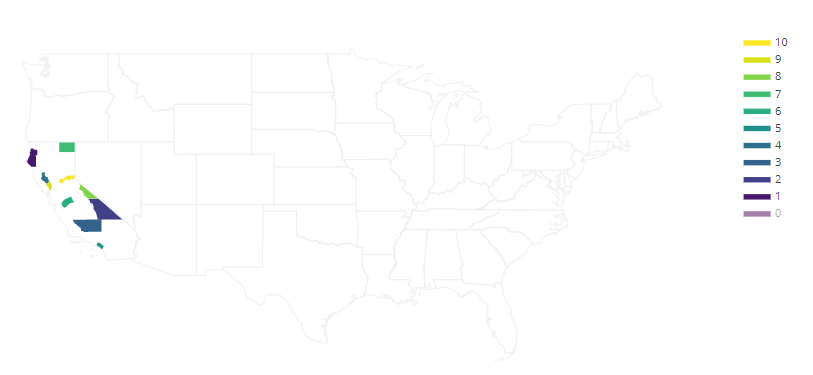GeeksforGeeks App
Open AppBrowser
Continue

# plotly.figure_factory.create_choropleth() function in Python

Plotly library of Python can be very useful for data visualization and understanding the data simply and easily.

## plotly.figure_factory.create_choropleth

A Choropleth Map is a map composed of colored polygons. It is used to represent spatial variations of a quantity.

Syntax: plotly.figure_factory.create_choropleth(fips, values, scope=[‘usa’], binning_endpoints=None, colorscale=None, order=None, legend_title=”, **layout_options)

Parameters:

fips ((list)) – it describes the list of FIPS values which correspond to the con catination of state and county ids.

values ((list)) – These are the values that will determine how the counties are colored.

scope ((list)) – it describes the list of states and/or states abbreviations. Fits all states in the camera tightly.

binning_endpoints ((list)) – it describes the ascending numbers which implicitly define real number intervals which are used as bins.

colorscale ((list)) – it is a list of colors with length equal to the number of categories of colors.

Example: Choropleth a few counties in California

## Python3

 `import` `plotly.figure_factory as ff`` ` `fips ``=` `[``'06021'``, ``'06023'``, ``'06027'``,``        ``'06029'``, ``'06033'``, ``'06059'``,``        ``'06047'``, ``'06049'``, ``'06051'``,``        ``'06055'``, ``'06061'``]``values ``=` `range``(``len``(fips))`` ` `fig ``=` `ff.create_choropleth(fips``=``fips, values``=``values)``fig.layout.template ``=` `None``fig.show()`

Output:My Personal Notes arrow_drop_up